# Algebra 1 : How to find percentage equivalent to a decimal

## Example Questions

← Previous 1 3 4 5

### Example Question #1 : How To Find Percentage Equivalent To A Decimal

Convert 0.4 to a percentage value.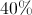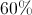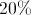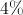Explanation:

To find the percentage, take the decimal and multiply it by 100. Another way is to move the decimal to the right two places.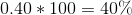### Example Question #2 : Decimals And Percentage

What decimal represents a quantity that has been reduced by?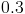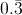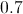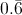Explanation:

If something is reduced by two thirds, the new quantity is: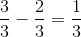This can be represented as a decimal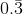.

### Example Question #1 : Decimals And Percentage

Convert 0.093 to a percent

none of these

0.93%

0.00093

93%

9.3%

9.3%

Explanation:

To transform 0.093 into a percent, move the decimal two digits to the right and then put in the '%' right after it.  In our case 0.093=9.3%

### Example Question #1 : How To Find Percentage Equivalent To A Decimal

Convert 1.03 to a percent.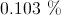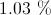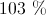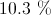Explanation:

To calculate a percent from a decimal, multiply by 100.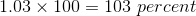### Example Question #1 : Decimals And Percentage

25 is what percent of 125?

.2%

25%

2%

None of the other answers are correct.

20%

20%

Explanation:

Write the statement as an equation and solve for: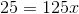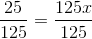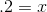Multiply the decimal by 100 to convert the answer to a percent: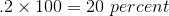### Example Question #1 : How To Find Percentage Equivalent To A Decimal

Convert decimals to percentages.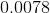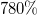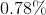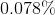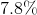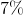Explanation:

To convert a decimal to percentage simply move the decimal point to the right two decimal places.

Thus,turns into.

In addition to this simple trick, one could also convert a decimal to a percentage by reading the decimal aloud.is read as "seventy-eight ten thousandths", this would look like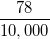. Since percentage is simply a representation of a part per hundred, if this fraction is reduced to a number over one hundred, we would get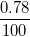, thus 78%.

### Example Question #1 : Decimals And Percentage

Convert decimal to percentage.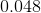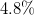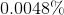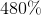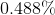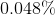Explanation:

Given, moving the decimal two places to the right result in.

Also, this decimal number read aloud is "forty-eight thousandths" , which is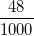. Since percentages are representations of a part per hundred,when the fraction is simplified by dividing both the numerator and denomintaor by ten to reduce the fraction to be out of one hundred, the numerator is left at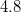, or.

### Example Question #1 : How To Find Percentage Equivalent To A Decimal

Convert decimal to percentage.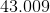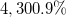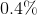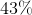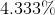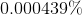Explanation:

Given, move the decimal point two places to the right, resulting in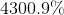.

Also,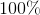is equivalent to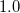. Thus,is roughly that, or, multipled by about 43. So we can expect our answer to be way over!

### Example Question #1 : How To Find Percentage Equivalent To A Decimal

Solve the problem and convert the answer to a percentage.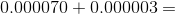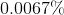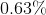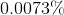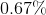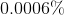Explanation: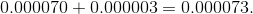To convert decimal to percentage, move the decimal to places to the right, resulting in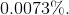\Alternatively, we could convert the decimals to percentages from the beginning.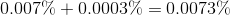### Example Question #1 : How To Find Percentage Equivalent To A Decimal

Convert decimal to percentage.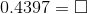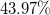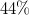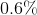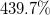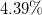Explanation: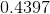to a percentage is solved by moving the decimal point two places to the right, resulting in.

This decimal read aloud reads "four thousand three hundred ninety seven ten thousandths", which in fraction form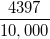, when reduced is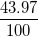, which is.

← Previous 1 3 4 5

### All Algebra 1 Resources